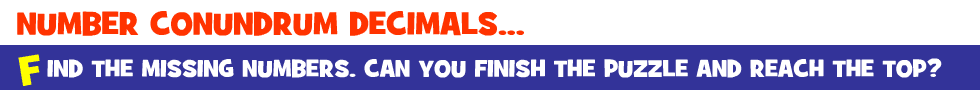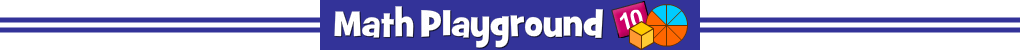Complete the number puzzle using decimal numbers.
The number in each block is the sum of the two numbers directly underneath.
Number Conundrum Decimals - Learning Connections
Essential Skills
Mental Math - work with fraction and decimal numbers

Common Core Connection for Grades 4+
Compare two fractions with different numerators and different denominators.
Use decimal notation for fractions with denominators 10 or 100.
Compare two decimals to hundredths by reasoning about their size.
Understand addition/subtraction of fractions as joining/separating parts of the same whole.
More Math Games to Play